# The Mixed Integer Linear Programming Solver

## Overview: MILP Solver

The OPTMODEL procedure provides a framework for specifying and solving mixed integer linear programs (MILPs). A standard mixed integer linear program has the formulation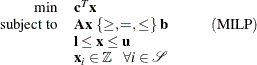where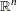is the vector of structural variables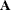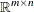is the matrix of technological coefficients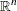is the vector of objective function coefficients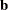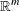is the vector of constraints right-hand sides (RHS)is the vector of lower bounds on variablesis the vector of upper bounds on variables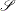is a nonempty subset of the set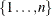of indices

The MILP solver, available in the OPTMODEL procedure, implements an linear-programming-based branch-and-bound algorithm. This divide-and-conquer approach attempts to solve the original problem by solving linear programming relaxations of a sequence of smaller subproblems. The MILP solver also implements advanced techniques such as presolving, generating cutting planes, and applying primal heuristics to improve the efficiency of the overall algorithm.

The MILP solver provides various control options and solution strategies. In particular, you can enable, disable, or set levels for the advanced techniques previously mentioned. It is also possible to input an incumbent solution; see the section Warm Start Option for details.